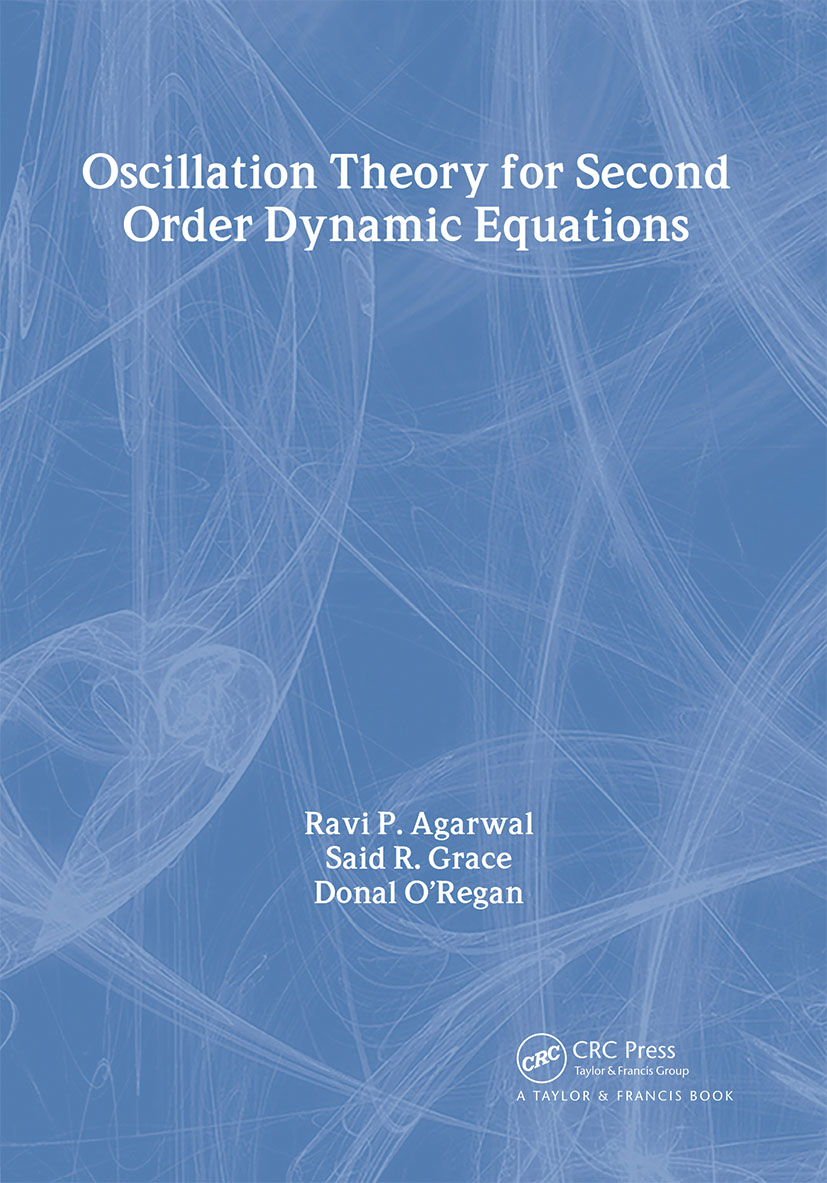# Oscillation Theory for Second Order Dynamic Equations

## 1st Edition

CRC Press

416 pages

##### Purchasing Options:\$ = USD
Hardback: 9780415300742
pub: 2002-11-21
SAVE ~\$29.00
Currently out of stock
\$145.00
\$116.00
x

FREE Standard Shipping!

### Description

The qualitative theory of dynamic equations is a rapidly developing area of research. In the last 50 years, the Oscillation Theory of ordinary, functional, neutral, partial and impulsive differential equations, and their discrete versions, has inspired many scholars. Hundreds of research papers have been published in every major mathematical journal. Many books deal exclusively with the oscillation of solutions of differential equations, but most of these books appeal only to researchers who already know the subject. In an effort to bring Oscillation Theory to a new and broader audience, the authors present a compact, but thorough, understanding of Oscillation Theory for second order differential equations. They include several examples throughout the text not only to illustrate the theory, but also to provide new direction.

### Reviews

"This book is very well organized … . [The material] is self-contained and consistent, and it is easy to follow the presentation. Only some background in calculus and differential equations is required. One definitely does not have to be a differential equations specialist in order to follow this well-written, compact, but thorough treatment."

- SIAM Review

Preliminaries. Introduction. Initial Value Problem, Oscillation and Nonoscillation. Continuability and Boundedness. Some Basic Results for Second Order Linear Ordinary Differential Equations. Some Useful Criteria for First Order. Some Useful Results from Analysis and Fixed Point Theorems. Notes and General Discussions. References. Oscillations of Differential Equations with Deviating Arguments. Oscillation Theorems (I). Oscillation Theorems (II). Comparison Theorems for Second Order Functional Differential Equations. Oscillation of Functional Equations with a Damping Term. Oscillation of Second Order Linear Delay Differential Equations. Oscillation of Forced Functional Differential Equations. Oscillation of Functional Equations with Damping and Forcing Terms. Necessary and Sufficient Conditions for the Oscillation of Forced Equations. Oscillation for Perturbed Differential Equations. Asymptotic Behavior of Oscillatory Solutions of Functional Equations. Notes and General Discussions. References. Oscillation of Neutral Functional Differential Equations. Oscillation of Nonlinear Neutral Equations. Oscillation of Neutral Equations with Damping. Oscillation of Forced Neutral Equations. Oscillation of Neutral Equations with Mixed Type. Necessary and Sufficient Conditions for Oscillations of Neutral Equations with Deviating Arguments. Comparison and Linearized Oscillation Theorems for Neutral Equations. Existence of Nonoscillatory Solutions of Neutral Delay Differential Equations. Asymptotic Behavior of Nonoscillatory Solutions of Neutral Nonlinear Delay Differential Equations. Notes and General Discussions. References. Conjugacy and Nonoscillation for Second Order Differential Equations. Conjugacy of Linear Second Order Ordinary Differential Equations. Nonoscillation Theorems. Integral Conditions and Nonoscillations. Notes and General Discussions. References. Oscillation of Impulsive Differential Equations. Oscillation Criteria for Impulsive Delay Differential Equations. Oscillation of Second Order Linear Differential Equations with Impulses. Notes and General Discussions. References. Subject Index with Deviating Arguments. Oscillation of Neutral Functional Differential Equations. Conjugacy and Nonoscillation for Second Order Differential Equations. Oscillation of Impulsive Differential Equations.

### Subject Categories

##### BISAC Subject Codes/Headings:
MAT007000
MATHEMATICS / Differential Equations
MAT037000
MATHEMATICS / Functional Analysis